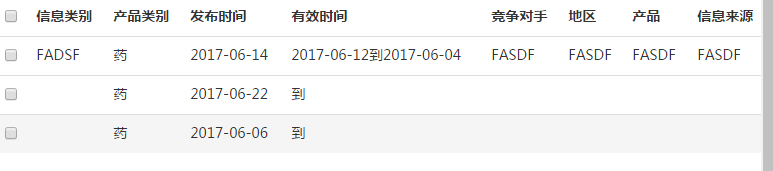data("id",id) 无法获取表格中<tr>所绑定的id值 10C
``````                                //绑定id值到tr
var tr="<tr>";
tr+="<td>";
tr+="<input type='checkbox' value='"+id+"' name='checkbox'/>";
tr+="</td>";
tr+="<td class='td_dep'>"+info_type+"</td>";
tr+="<td class='td_dep'>"+type+"</td>";
tr+="<td class='td_dep'>"+release1+"</td>";
tr+="<td class='td_dep'>"+effective_s+"到"+effective_o+"</td>";
tr+="<td class='td_dep'>"+compete+"</td>";
tr+="<td class='td_dep'>"+region+"</td>";
tr+="<td class='td_dep'>"+product+"</td>";
tr+="<td class='td_dep'>"+source+"</td>";
tr+="</tr>";
var \$tr=\$(tr);
\$tr.data("id",id);
console.log(\$tr.data("id"));

//获取从tr中获取id值
\$("#table_content").on("click",".td_dep",function(){

//获取绑定 的ID值
var \$tr=\$(this).parent();
var id=\$tr.data("id");
console.log(id);

各位大神，为什么获取id值总是提示undifine,需要怎么解决呢

``````6个回答

`````` <script type="text/javascript" src="https://cdn.bootcss.com/jquery/1.7.1/jquery.min.js"></script>
<table id="tb"></table>
<script type="text/javascript">
\$tr=\$('<tr><td class="td_dep">11</td></tr>')
\$tr.data('id', 123);
console.log(\$tr.data('id'));
\$tr.appendTo('#tb');
\$('#tb').on('click', '.td_dep', function () { alert(\$(this).parent().data('id')) })
</script>
``````

value='"+id+"' 你要不试试** id='"+id+"' **powerApower checkbox获取数据正常，不正常的是我将id值绑定到tr上无法获取
3 年多之前 回复

\$(":input[name='checkbox']").val()这样试试？我不懂你那样写是什么意思powerApower 回复meicuojiushiwoi: 是的，获取一行数据
3 年多之前 回复meicuojiushiwoi 收回我的话，你应该是想获取一行的数据吧
3 年多之前 回复

`````` \$('#tb').on('click', '.td_dep', function () {
var \$tr=\$(this).parent();
var id =\$tr.find("input[type=checkbox]").val();

}试试吧
``````